• FIR设计-基于Matlab的FIR低通滤波器的设计与仿真.pdf 基于Matlab的FIR低通滤波器的设计与仿真.pdf FIR设计 FIR滤波器设计与仿真。
• 基于MATLAB的设计fir低通滤波器，采用方法是频率抽样法
• 基于MATLAB的FIR低通滤波器，newfft是自己编写的，也可以直接调用MATLAB内部的fft程序，runfir文件作为一个运行的实例，数字信号处理方面可能用得到。
• 简述用MATLAB设计低通滤波器的程序，主要针对MATLAB初学者!
• 匿名用户1级2011-05-20 回答%所希望频率响应在0~0.25*pi之间为1，...%首先需要理解实际的滤波器是没有办法做到理想状态。如果你要理想状态话，%就直接就是矩形窗序列乘序列就OK， 矩形窗只能达到20.9dB 衰减...
匿名用户1级2011-05-20 回答%所希望的频率响应在0~0.25*pi之间为1，在0.25*pi~pi之间为0，对h(n)加窗截断，%选择矩形窗和任意一种其他窗口，每种窗口长度分别为M=10、20、40三种情况，%对每种情况要进行性能好坏的分析。%首先需要理解实际的滤波器是没有办法做到理想状态的。如果你要理想状态的话，%就直接就是矩形窗序列乘序列就OK，  矩形窗只能达到20.9dB 的衰减%但是实际的加窗构成的滤波器，必然会存在波纹，和过渡带 滤波器的衰减指标主要%是由阻带衰减确定的 不妨设 阻带衰减为 50dB  那么要达到这个指标，通过查表，%可以知道-必须是hamming window(54.5dB) 或 blackman window(75.3dB)%下面是加窗的低通FIR滤波器clearclc;wc=0.25*pi;wdelta=wc-2*wc/3;           %设定过渡带宽度M=ceil(3.32*pi/wdelta) ;       %窗口长度 这里计算合理的窗长N1=2*M+1;winlp=hamming(N1);Flp=fir1(N1-1,wc/pi,winlp);freqz(Flp,1,512);title('Frequency & Phase Response ');% 下面就是按你的要求啦，窗长M=10、20、40M=[10,20,40];N=2*M+1;% M=10figure(2);Win0=hamming(N(1));FLP0=fir1(N(1)-1,wc/pi,Win0);freqz(FLP0,1,512);title('Frequency & Phase Response M=10');% M=20figure(3);Win1=hamming(N(2));FLP1=fir1(N(2)-1,wc/pi,Win1);freqz(FLP1,1,512);title('Frequency & Phase Response M=20');% M=30figure(4);Win2=hamming(N(3));FLP2=fir1(N(3)-1,wc/pi,Win2);freqz(FLP2,1,512);title('Frequency & Phase Response M=30');% 至于矩形窗 设 20dB衰减figure(5)M0=ceil(0.92*pi/wdelta) ;       %窗口长度 这里计算合理的窗长N0=2*M0+1;ww=boxcar(N0);fflp=fir1(N0-1,wc/pi,ww);freqz(fflp,1,512);title('Frequency & Phase Response Rectangular');
展开全文• 使用MATLAB设计FIR低通滤波器 ** 关于现代通信原理作业。 https://blog.csdn.net/tanghonghanhaoli/article/details/100533581 这是老师给出提示，里面有比较详细讲解，下面写一些我想法。 1. 为什么要设计低...
**
使用MATLAB设计FIR低通滤波器
**
关于现代通信原理作业。
https://blog.csdn.net/tanghonghanhaoli/article/details/100533581
这是老师给出的提示，里面有比较详细的讲解，下面写一些我的想法。
1. 为什么要设计低通滤波器
我们可以看到例子中给的m(t)在时域中已经是物理可实现的信号了，但是在频域上看这个信号的频谱是无限宽的，显然这用计算机是难以处理的，加上Sa信号旁瓣的影响并不是很大，所以我们考虑滤除旁瓣，即使用低通滤波器。
2. 关于如何设计低通滤波器的理论分析
对于例题中是想保留Sa信号±10Hz内的频率分量，即需要设计一个频率范围在±10Hz的低通滤波器h(t)，也就是频域为一个方波信号H(f)。由信号与系统的知识可得，频域方波，时域则为Sa信号。时域h(t)如果为标准的Sa函数，则在t<0的时候，h(t)还会有值，这明显是物理不可实现的，所以这里考虑将h(t)进行一定的时移。
注意：这里并不会影响滤波器的功能，因为时域平移对应频域的相移，但是幅度没有改变，所以即使时域平移了，频域的幅频特性并不会改变，依然是在±10Hz通过信号。
3. 关于MATLAB的Filter Designer
在MATLAB的Filter Designer中就将中心移到了(N/2)*Ts，N就是对h(t)的抽样点个数。时域抽样点越多，对应的时域波形就越接近Sa信号，频域就越接近方波，即越接近我们要的低通滤波器。同时，如果抽样频率Fs不变，则Ts也不会改变，抽样点N增加，那么中心平移得就更多了，即时延就更多了；换个角度理解，抽样点更多，抽样时间间隔Ts不变，也就意味着对更高频的旁瓣也会被采样，则被采样的Sa信号更宽了，占用的时间也更多了，也能想象时延会更大。
但是在MATLAB中，N好像是由系统根据你设置的参数自动匹配的。我没有看到设置抽样个数N的选项，只有设置抽样频率Fs的选项，N会根据你设置的Fs进行自动匹配，就是设置完参数后点击Design Filter后，左上角看到的order的数值。Fs增加，即Ts减小，N会随之增加，在MATLAB中，我们可以看到两者的变化速率是一致的，所以中心始终在一个位置。（所以我在想是不是MATLAB关于Design Filter的系统设置就是只采样主瓣和相邻几个旁瓣的数值，这样也确实已经很接近一个Sa信号了，更高频的旁瓣其实幅度很小，近似为0应该也不会带来太多误差）本人学生，难免学艺不精，若有疏漏错误之处，敬请指正!


展开全文• 本程序是用matlab实现的FIR低通滤波器，通过加载一段音频文件，进行滤波处理，最后又回放滤波器后的音频文件…… 可以通过听觉上区分滤波前后的差别，更好的方式当然是通过观察滤波前后的频谱来进行验证……
• matlab实现单声道立体声信号的fir低通滤波，使用窗函数法，并将输入数据进行输出，供硬件仿真使用。
• 基于FPGA低通滤波器FIR的设计1 滤波器特征参数介绍图1 低通滤波器特征参数 如图1所示，低通滤波器的通带截止频率为ωp ,通带容限为α1，阻带截止频率为ωs,阻带容限为α2。通带定义为|ω|≤ωp ,过渡带定义为ωp2 ...


基于FPGA低通滤波器FIR的设计1 滤波器的特征参数介绍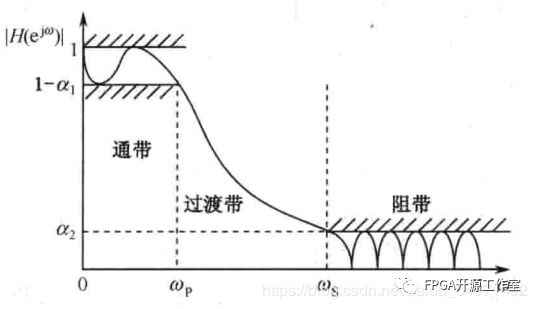图1 低通滤波器特征参数       如图1所示，低通滤波器的通带截止频率为ωp ,通带容限为α1，阻带截止频率为ωs,阻带容限为α2。通带定义为|ω|≤ωp ,过渡带定义为ωp2 设计目标      要求：使用fir滤波器设计一个滤波器系数为15阶的低通滤波器，通带截止频率为1KHZ，采样频率为44.1khz。3 matlab的设计验证close allclear allclc%设计一个低通滤波器 采样频率为44.1khz, 截止频率为1khzFS = 44100; %HZfc = 1000;N  = 15;Q  = 16;%以采样频率的一半，对频率进行归一化处理wn_lpf=fc*2/FS;%采用fir1函数设计FIR滤波器b_lpf=fir1(N-1,wn_lpf);%滤波系数进行量化b_16=round(b_lpf/max(abs(b_lpf))*(2^(Q-1)-1));%求滤波器的幅频响应m_lpf=20*log(abs(fft(b_lpf)))/log(10);b16_lpf=20*log(abs(fft(b_16)))/log(10);%设置幅频响应的横从标单位为Hzx_f=[0:(FS/length(m_lpf)):FS/2];  %绘制单位脉冲响应subplot(221);stem(b_lpf);xlabel('n');ylabel('h(n)');title('低通滤波器的单位脉冲响应','fontsize',8);subplot(222);stem(b_16);xlabel('n');ylabel('h(n)');title('低通滤波器的单位脉冲响应','fontsize',8);%绘制幅频响应曲线subplot(223);plot(x_f,m_lpf(1:length(x_f)));xlabel('频率(Hz)','fontsize',8);ylabel('幅度(dB)','fontsize',8);title('低通滤波器的幅频响应','fontsize',8);subplot(224);plot(x_f,b16_lpf(1:length(x_f)));xlabel('频率(Hz)','fontsize',8);ylabel('幅度(dB)','fontsize',8);title('低通滤波器的幅频响应','fontsize',8);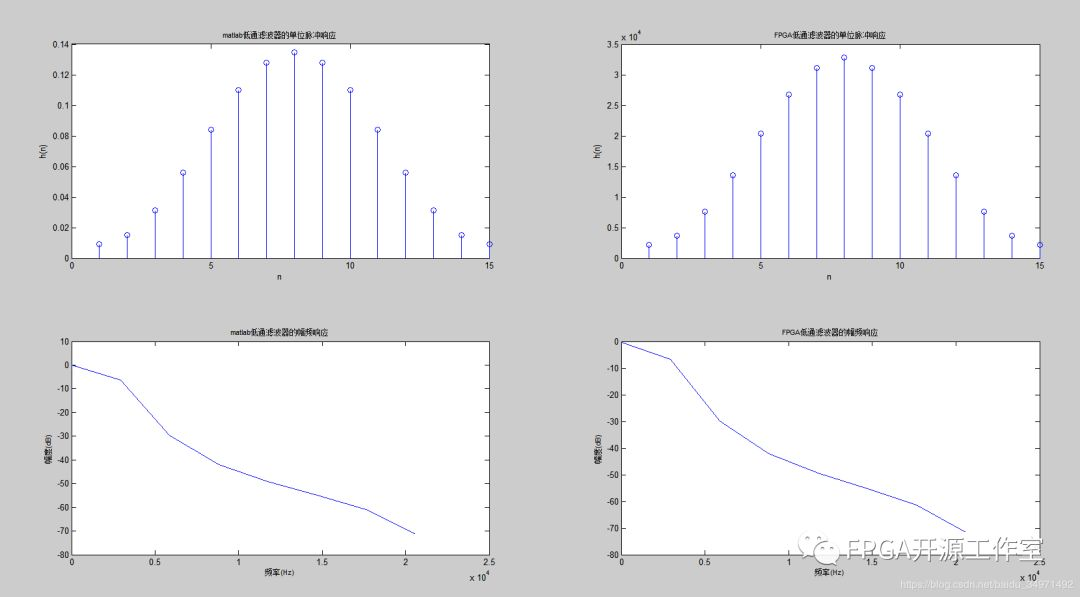图2 低通滤波器的matlab单位脉冲响应和幅频响应4 FPGA信号发生器为了验证低通滤波器，我们设计了测试验证的sin信号发生器。wire [17:0] kfreq= (k<2048)?18’h00333:18’h00FFF;//1khz-5khz产生1khz和5khz的sin波。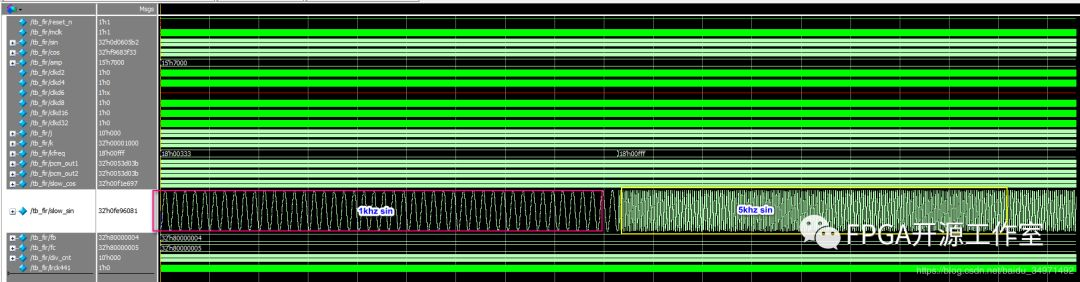图3 信号发生器产生1khz和5khz的sin测试源测试源的FFT分析：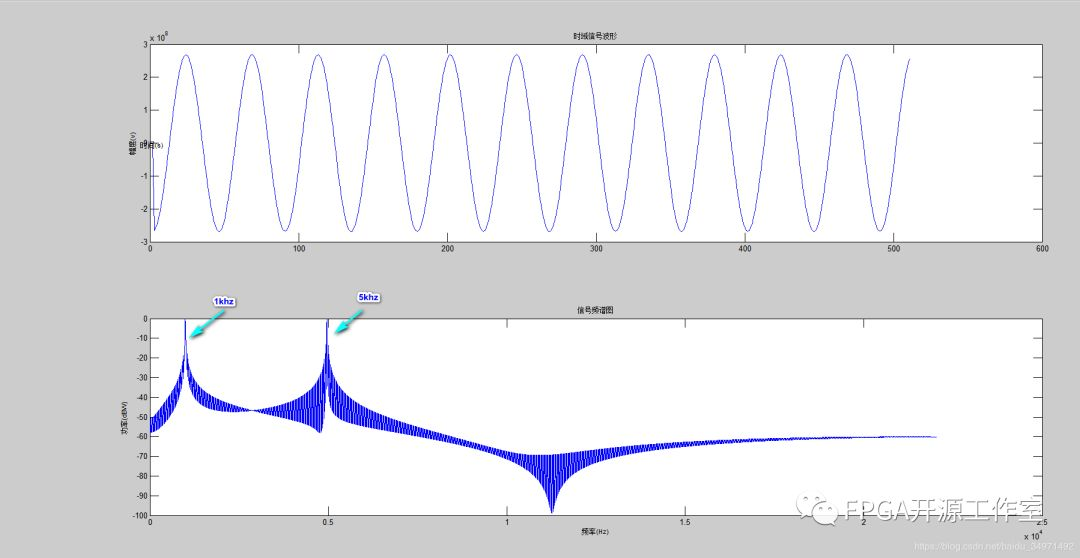图4 测试源数据的matlab分析      由图4的频域图可知，测试源产生了1khz和5khz的sin。5 FPGA的fir设计      Fir滤波器verilog代码设计采取全并行模式。timescale 1ps/1psmodule fir1(           input mclk,//45.1584MHZ	   input reset_n,	   input signed[31:0] pcm_in,	   output signed[31:0] pcm_out);localparam LAST_CYCLE = 1023;reg [9:0] i;reg signed [31:0] pcm1,pcm2,pcm3,pcm4,pcm5,pcm6,pcm7,pcm8,pcm9,pcm10,pcm11,pcm12,pcm13,pcm14,pcm15;reg signed [47:0] pcm1_out,pcm2_out,pcm3_out,pcm4_out,pcm5_out,pcm6_out,pcm7_out,pcm8_out,pcm9_out,pcm10_out,pcm11_out,pcm12_out,pcm13_out,pcm14_out,pcm15_out;reg signed [51:0] pcm_r;wire signed [15:0] coeff1,coeff2,coeff3,coeff4,coeff5,coeff6,coeff7,coeff8,coeff9,coeff10,coeff11,coeff12,coeff13,coeff14,coeff15;assign coeff1 = 2208;assign coeff2 = 3631;assign coeff3 = 7612;assign coeff4 = 13576;assign coeff5 = 20413;assign coeff6 = 26727;assign coeff7 = 31169;assign coeff8 = 32767;assign coeff9 = 31169;assign coeff10 = 26727;assign coeff11 = 20413;assign coeff12 = 13576;assign coeff13 = 7612;assign coeff14 = 3631;assign coeff15 = 2208;assign pcm_out = pcm_r[51:20];always @(posedge mclk or negedge reset_n) beginif(!reset_n) begin    i<= 0;	pcm1<=0;	pcm2<=0;	pcm3<=0;	pcm4<=0;	pcm5<=0;	pcm6<=0;	pcm7<=0;	pcm8<=0;	pcm9<=0;	pcm10<=0;	pcm11<=0;	pcm12<=0;	pcm13<=0;	pcm14<=0;	pcm15<=0;    pcm1_out<=0;	pcm2_out<=0;	pcm3_out<=0;	pcm4_out<=0;	pcm5_out<=0;	pcm6_out<=0;	pcm7_out<=0;	pcm8_out<=0;	pcm9_out<=0;	pcm10_out<=0;	pcm11_out<=0;	pcm12_out<=0;	pcm13_out<=0;	pcm14_out<=0;	pcm15_out<=0;	pcm_r<= 0;  endelse begin    i <= i + 1;if(i == 0) begin	  pcm1<=pcm_in;	  pcm2<=pcm1;	  pcm3<=pcm2;	  pcm4<=pcm3;	  pcm5<=pcm4;	  pcm6<=pcm5;	  pcm7<=pcm6;	  pcm8<=pcm7;	  pcm9<=pcm8;	  pcm10<=pcm9;	  pcm11<=pcm10;	  pcm12<=pcm11;	  pcm13<=pcm12;	  pcm14<=pcm13;	  pcm15<=pcm14;	endif(i==1) begin    	pcm1_out <= pcm1*coeff1;	    pcm2_out <= pcm2*coeff2;	    pcm3_out <= pcm3*coeff3;	    pcm4_out <= pcm4*coeff4;	    pcm5_out <= pcm5*coeff5;	    pcm6_out <= pcm6*coeff6;	    pcm7_out <= pcm7*coeff7;	    pcm8_out <= pcm8*coeff8;	    pcm9_out <= pcm9*coeff9;	    pcm10_out <= pcm10*coeff10;	    pcm11_out <= pcm11*coeff11;	    pcm12_out <= pcm12*coeff12;	    pcm13_out <= pcm13*coeff13;	    pcm14_out <= pcm14*coeff14;	    pcm15_out <= pcm15*coeff15;	endif(i==2) pcm_r<= pcm1_out +pcm2_out+pcm3_out+pcm4_out+pcm5_out+pcm6_out+pcm7_out+pcm8_out+pcm9_out+pcm10_out+pcm11_out+pcm12_out+pcm13_out+pcm14_out+pcm15_out;  endendendmodule     fpga实验modelsim仿真结果，从图5来看1khz几乎保持不变，而5khz波形幅度上被抑制了很多。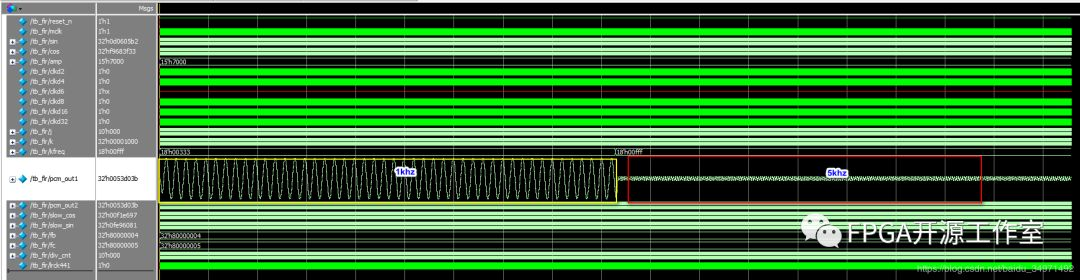图5 modelsim fir实验结果时域波形Matlab fft分析：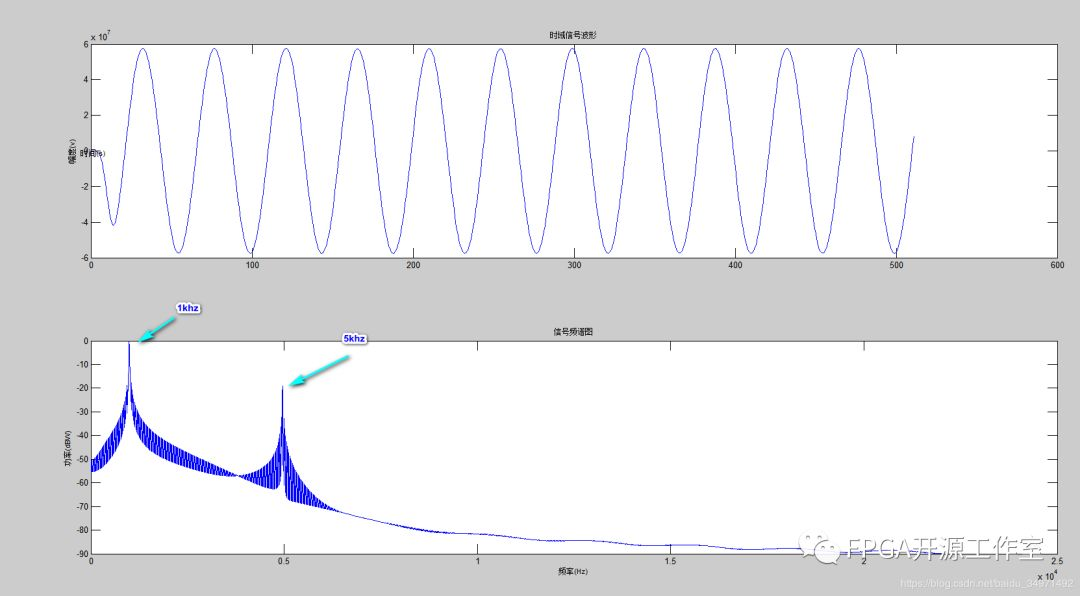图6 matlab分析实验结果      由图2和图6对比，5khz经过滤波后的功率减小了20DB，图2与图5基本一致，fir的低通滤波器全并行设计成功。DSP往期音频总线I2S协议信号的产生信号的基本概念基于LUT的DDS的设计更多精彩推荐，请关注我们万水千山总是情，点个 “好看” 行不行！！！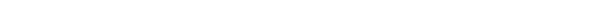展开全文• 基于MATLAB的FIR数字低通滤波器分析和设计
• 充分利用有限冲击响应数字滤波器（Finite Impulse Response digital filter ，FIR）系数对称特性，借助于MATLAB语言和现场可编程门阵列（FPGA）实现了一种高效的低通滤波器。设计过程中通过简化VHDL语言编写程序...
• 1 绪 论 1.1 引言 滤波技术是信号分析信号处理技术中重要分支无论是信号获取传输还是信号处理转换都离不开滤波技术滤波技术对信号安全可靠和有效灵活传递至关重要1 在电子系统中由于滤波器的好坏直接影响...
• matlab 一个简单的FIR低通滤波器设计例子 先直接放代码跟结果 %假设有一个3hz的信号，由于某种原因带有一些高频噪声，需要将其滤除掉 close all; clear all; load lowpass_fir; %fs=20 fc=6的32阶低通滤波器汉明窗 ...
matlab 一个简单的FIR低通滤波器设计例子
先直接放代码跟结果
%假设有一个3hz的信号，由于某种原因带有一些高频噪声，需要将其滤除掉
close all;
clear all;

fs = 20;  %采样率
T0 = 10;  %信号时长10s
F0 = 1/T0; %频率分辨率
N = fs*T0; %采样点数
t = (0:N-1)/fs;  %时间轴坐标
f = (0:F0:fs/2-F0);  %频率轴坐标

x = sin(2*pi*3*t)+1.2*sin(2*pi*7*t)+0.7*sin(2*pi*8*t)+0.2*randn(size(t));  %模拟信号
subplot(2,2,1);
plot(t(1:100),x(1:100));

fx = abs(fft(x))*(2/N); %2/N中的N很好理解，2是因为matlab的FFT把负频也算上了，只分析正频需要加倍
subplot(2,2,3);
plot(f,fx(1:N/2));

y = filter(b_fir,1,x);
subplot(2,2,2);
plot(t(1:100),y(1:100));

fy = abs(fft(y))*(2/N);
subplot(2,2,4);
plot(f,fy(1:N/2));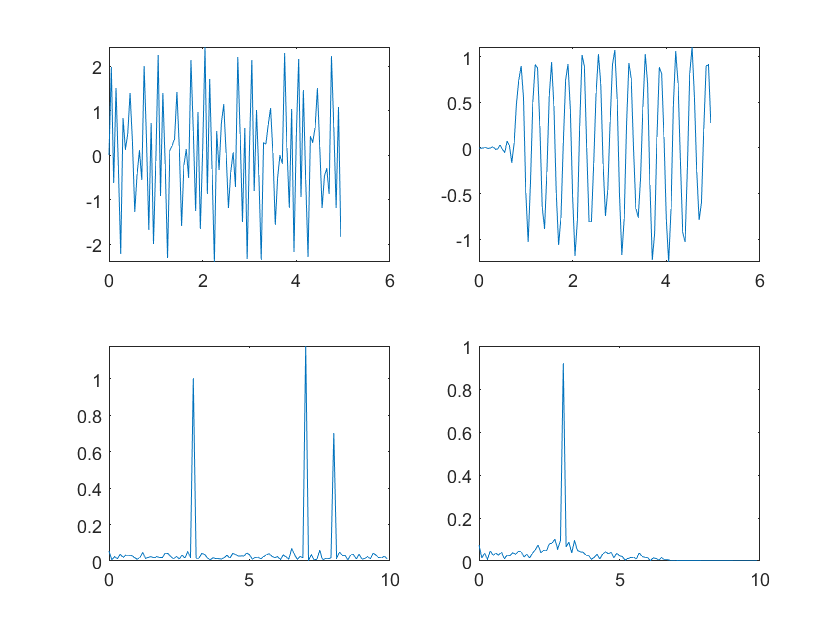1.输出有延迟，FIR滤波器的特性，阶数越大延迟越高，延迟为（N-1)/2,
2.窗函数法不可避免带来一定的频谱泄露，所以输出在3hz幅度变小，附近的频率分量增大
要有基本的数信理论才能看懂，最重要的是两点性质
1.观测时长为T0，则频率分辨率为F0=1/T0
2.采样频率为fs，则最大观测频率为fs
这样才能将坐标系对应上
matlab的滤波器设计如下，需要将滤波器系数导出这样方便使用，有了系数就可以用C语言去实现了。对于入门来说，用什么窗，阶数是多少就随便了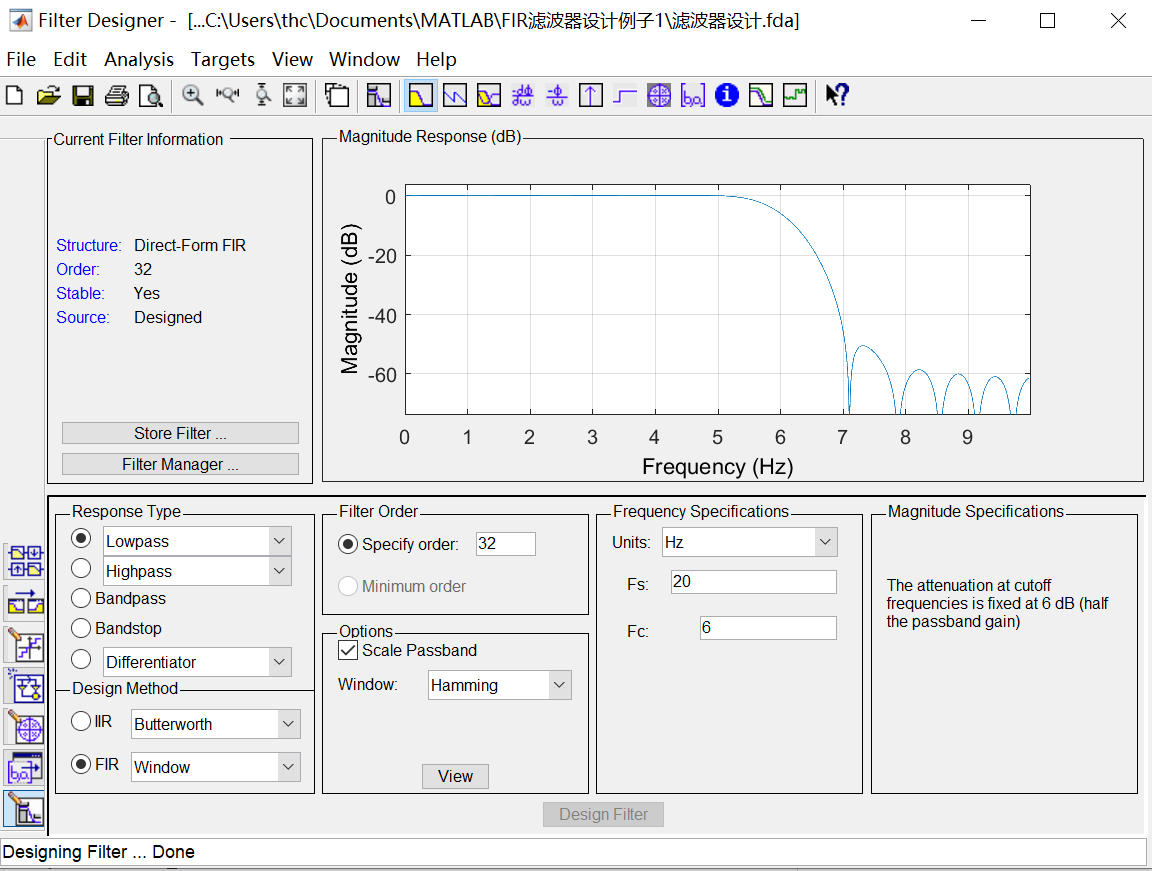`
展开全文• 应用matlab进行编程设计FIR低通滤波器的设计
• 数字低通滤波器FIR，用MATLAB仿真，可以运行
• Matlab 实现振动信号低通滤波 附件 txt 中的数字是一个实测振动信号采样频率为 5000Hz试设计一个长度 为 M=32 的FIR 低通滤波器截止频率为 600Hz用此滤波器对此信号进行滤波 要求 1 计算数字截止频率 2 给出滤波器...
• 按照所通过信号频率不同分为：低通滤波器-允许信号中低频或直流分量通过，抑制高频分量或干扰和噪声；高通滤波器-允许信号中高频分量通过，抑制低频或直流分量；带通滤波器-允许一定频段信号通过，抑制低于...
• 滤波器是电阻抗成像（Electrical Impedance Tomography, EIT）硬件...为降低电阻抗成像硬件系统成本，使用最佳方法（即切比雪夫最佳一致逼近法）设计FIR低通滤波器，通过Matlab仿真验证后在ARM处理器CortexM3上实现。
• 一、摘要前面一篇文章介绍了通过FDATool工具箱实现滤波器设计，见“二、实验平台Matlab7.1三、实验原理以低通滤波器为例，其常用设计指标有：通带边缘频率fp(数字频率为Ωp)阻带边缘频率fst(数字频率为Ωst)通带...
• matlab开发-低通滤波器设计。基于约束二次规划（QP）低通FIR数字微分器（LPFIRDD）设计。
• matlab中用汉明窗设计低通滤波器，主要用于心电信号滤波，可通过直接调节参数进行截止频率修改。方便好用
• %设计低通滤波器：[N,Wc]=buttord()%估算得到Butterworth低通滤波器的最小阶数N和3dB截止频率Wc[a,b]=butter(N,Wc); %设计Butterworth低通滤波器[h,f]=freqz(); %求数字低通滤波器的频率响应figure(2); % 打开窗口2...
• %设计FIR数字低通滤波器，截止频率为pi/4，在不同窗口长度（N=15，N=33）下，分别求出 %h(n),通过幅频特性和相频特性，观察3dB带宽和20dB带宽，总结窗口长度N对滤波特性影响。 close all; clc; clear; N=15; N1=N...
• （一） 设计一个低通滤波器  采样频率 Fs = 50Hz Fpass = 10Hz Fstop = 20Hz 打开matlab，键入fdatool后，会弹出该工具对话框。然后按照下图设置相关参数，参数列表中Fs应该是采样频率，Fc是截止频率，即...
• FIR滤波器很多工科出身的人都不会陌生，在...本文主要介绍MATLAB最常用的FIR滤波器设计方法之窗函数法。其他的方法将在另一章中介绍。窗函数法是一种基础且普遍应用的FIR滤波器设计方法。首先需要根据性能指标(如...
• 一、实验目的(1)综合应用信号频谱分析和数字滤波器设计知识，实现信号滤波。(2)比较分析干扰信号加入前、后频谱有何区别，...二、实验原理(1) FIR数字滤波器的设计原理：FIR数字滤波器总是稳定系统，且可以......

# matlab的fir低通滤波器matlab 订阅